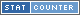# Multiplication

Multiplication also refered to as times is denoted with either the "x" symbol or "*" (typically when written) or astric symbol "*" astric symbol when used in computer languages or code.

When multiplying it is the first number and add it together as many times as their is the following number.

## Examples

Here are some link examples of multiplying.

The above are just a few examples of multiplication, use the search at the top to find more.